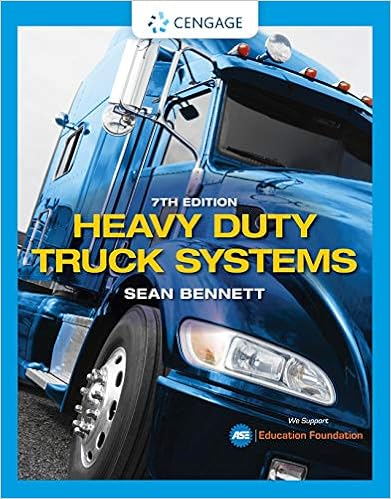Consider this circuit 3 determine the thevenin

• Test Prep
• 17
• 100% (5) 5 out of 5 people found this document helpful

This preview shows page 3 - 7 out of 17 pages.

We have textbook solutions for you!
The document you are viewing contains questions related to this textbook.The document you are viewing contains questions related to this textbook.
Chapter 5 / Exercise 21
Heavy Duty Truck Systems
BennettExpert Verified
3-4 . Consider this circuit: 3. Determine the Thevenin resistance, R Th , as seen by the load resistor R L . A. R Th = 1 kΩ B. R Th = 6 C. R Th = 9 D. R Th = 3 E. R Th = 2 kΩ kΩ kΩ kΩ
4. Determine the short circuit current I sc (in the direction from the top node to the bottom node), produced when R L is replaced by a short.
We have textbook solutions for you!
The document you are viewing contains questions related to this textbook.The document you are viewing contains questions related to this textbook.
Chapter 5 / Exercise 21
Heavy Duty Truck Systems
BennettExpert Verified
5. The I – V characteristic of an unknown linear circuit is shown. Determine which of the equivalent models shown represents the linear circuit.
6. Determine the output voltage V out as a linear combination of the sources I 1 and V 2 . 1 1 2 2 2
7. Determine the Thevenin equivalent circuit as seen by the voltage source V 1 . Use the idealized model of the op-amp and assume that the op amp is operating in its linear region. kΩ
8. Assume that the value of the load resistor, R L , allows maximum power transfer to the load resistor. Determine the power, P L , dissipated by the load resistor. A. P L = 20 mW B. P L = 80 mW C. P L = 10 mW D. P L = 160 mW E. P L = 40 mW
•••